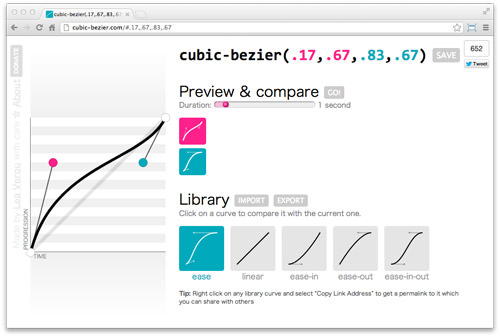# A Look Into: Cubic-bezier in CSS3 Transition

CSS3 Transition is one of the great additions in CSS3. It allows us to apply transitional changing effects to CSS rules. If you are already familiar with the syntax, you will see that we need to specify the timing function or the transition speed.

There are four predefined timing functions we can use, they are `ease`, `ease-in`, `ease-out`, `ease-in-out` and `linear`.

But, one thing that most people overlook in these functions is that they are essentially based on the Bezier curve.

### Bezier Curve

The Bezier curve is a commonly used curve model in computer graphics. If you have been working with Vector Graphic Editors like Adobe Illustrator or Inkscape, you will find this curve model when you are drawing with the pen tool. This curve is formed with four points as illustrated in the following figure:Thus, in CSS3 Transition, the cubic-bezier function is defined within this syntax:

```cubic-bezier(P0,P1,P2,P3);
```

It is worth noting that each point in this Cubic-bezier only allows the values from 0 to 1. Alright, let’s return to the timing functions in CSS3 transition we have mentioned earlier and see how they are actually formed in the Cubic-bezier curve.

ease, this is the default timing function in CSS3 Transition and in cubic-bezier format it can be translated as follows:

```cubic-bezier(0.25,0.1,0.25,1);
```

linear, using linear timing function, the speed will be steady from start to end and in cubic-bezier format it is translated, as follows:

```cubic-bezier(0,0,1,1)
```

ease-in, using this timing function, the animation will start slowly and then gain more acceleration and get steady until the end of the duration. In cubic-bezier format it can be translated into:

```cubic-bezier(0.42, 0.0, 1.0, 1.0)
```

ease-out, this timing function simply does the opposite to ease-in. The speed will start steady and fast, then just about the end of duration it will slow down. In the cubic-bezier format, this can be translated into:

```cubic-bezier(0.0, 0.0, 0.58, 1.0)
```

ease-in-out, this timing function is simply a combination between ease-in and ease-out, the animation starts slow, accelerate in the middle, then ends slow. In cubic-bezier this can be translated, as follows.

```cubic-bezier(0.42, 0.0, 0.58, 1.0)
```

### Creating Custom Speed

Using Cubic-bezier function, we are able to create custom speed. But one of the main constraint of defining animation speed with Cubic-bezier function is that it is not that intuitive, and we cannot see instantly how the speed is moving. Luckily, there is one tool that we can use to help us out, named cubic-bezier.com.This tool is created by Lea Verou, also known as the CSS Guru. It has the Bezier curve that you can move around, and it will instantly generate the values for you. We can also show the transition movement and compare the speed.

This example shows how we can utilize cubic-bezier function to set a rocket speed.You can view the demo and download the source from these links. We hope you enjoyed it.